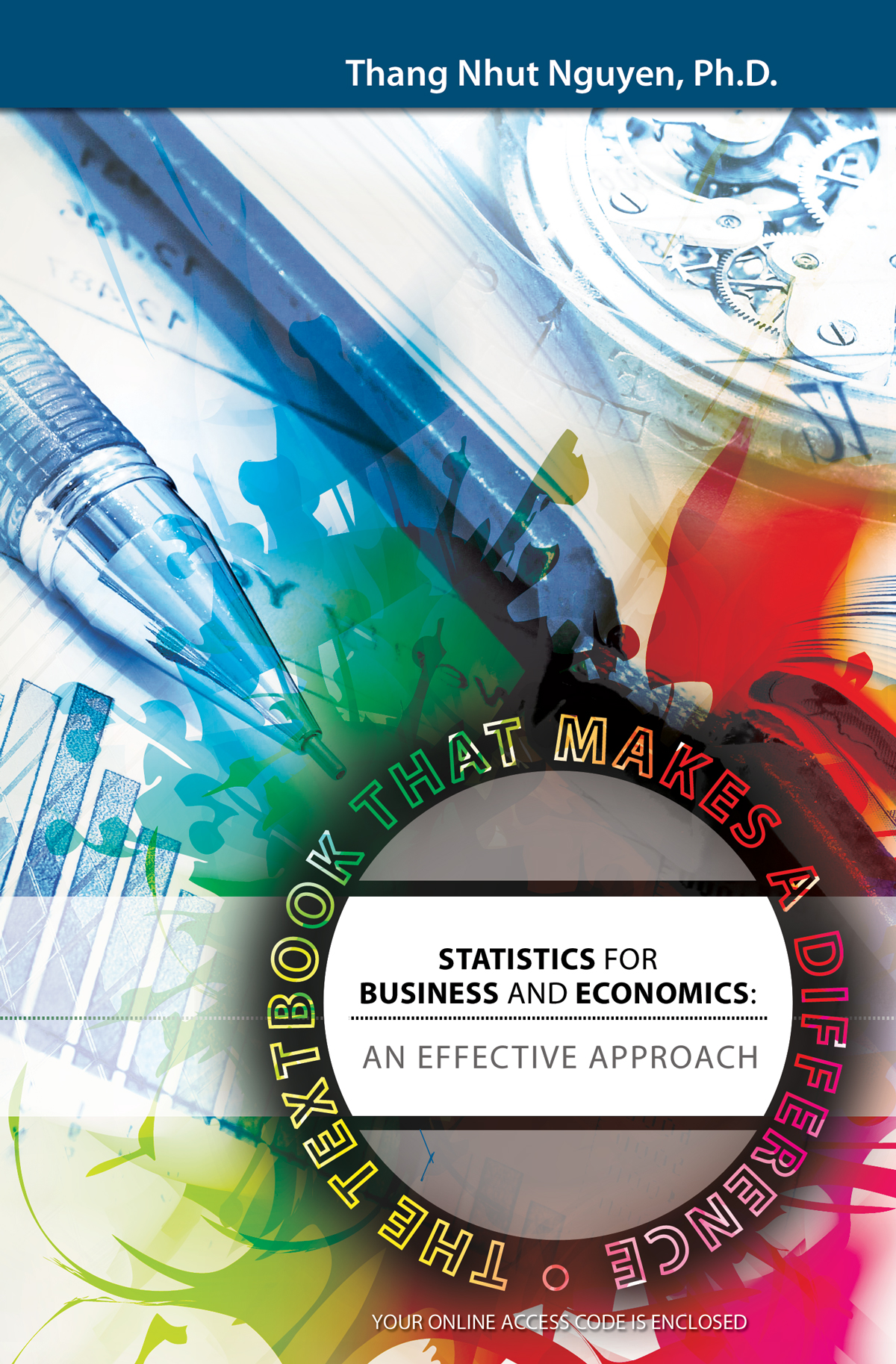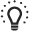## Essentials of Statistics for Business and Economics: The 2-Pass+ Approach - The Textbook that Makes a Difference### Overview ofEssentials of Statistics for Business and Economics: The 2-Pass+ Approach - The Textbook that Makes a Difference.

Chapter 0: Core Concepts and Core Techniques

Chapter 1: Data, Information, Knowledge and Decision Making

Chapter 2: Measures and Presentations

Chapter 3: Basic Mathematics of Probability

Chapter 4: Discrete Probability Distributions

Chapter 5: Continuous Probability Distributions

Chapter 6: Sampling Distribution

Chapter 7: Interval Estimation

Chapter 8: Hypothesis Testing

Chapter 9: Regression models

Chapter 10: Extension to inference about population variance

Chapter 11: Extension to Statistical tests using Chi-square tests

Chapter 12: Extension to Analysis of Variance using F-tests